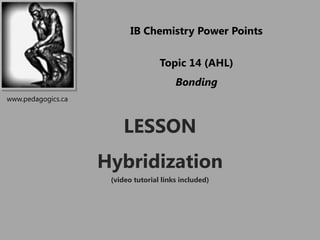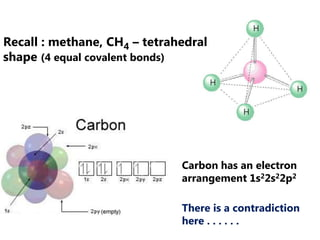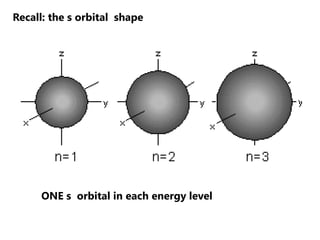Successfully reported this slideshow.

# 2012 Orbital Hybrization, Sigma and Pi Bonds

17

Share×
1 of 25
1 of 25

# 2012 Orbital Hybrization, Sigma and Pi Bonds

17

Share

## More Related Content

### Related Books

Free with a 30 day trial from Scribd

See all

### Related Audiobooks

Free with a 30 day trial from Scribd

See all

### 2012 Orbital Hybrization, Sigma and Pi Bonds

1. 1. IB Chemistry Power Points Topic 14 (AHL) Bonding www.pedagogics.ca LESSON Hybridization (video tutorial links included)
2. 2. Recall : methane, CH4 – tetrahedral shape (4 equal covalent bonds) Carbon has an electron arrangement 1s22s22p2 There is a contradiction here . . . . . .
3. 3. Recall: the s orbital shape ONE s orbital in each energy level
4. 4. Recall: the p orbital shape THREE p orbitals in each energy level (beginning n = 2)
5. 5. Carbon’s valence shell - 2nd energy level orbitals The 4 orbitals are oriented like so: Problem #1 This is not a tetrahedral shape. The 3 p orbitals are at 900 to each other and the s orbital is “non-directional”
6. 6. Carbon ground state electron configuration What is the expected orbital notation of carbon in its ground state? Problem #2? Only 2 electrons appear to be available for bonding
7. 7. Solution – require 4 unpaired electrons Perhaps carbon “promotes” one of its 2s electrons… …to the empty 2p orbital.
8. 8. BUT. . . this would mean that three of the bonds in a methane molecule would be identical, But what about the fourth bond…? Must have a lower bond energy This was not observed by chemists
9. 9. “hybridization” theory - What if the s orbital is combined with three p orbitals to create four equal hybrid orbitals? “hybridized” These new orbitals have slightly MORE energy than the 2s orbital… and slightly LESS energy than the 2p orbitals. … But all 4 hybrid orbitals have equal energy.
10. 10. Since the 4 hybrid orbitals result from a combination of 1 s and 3 p orbitals, these hybrids are termed sp3 orbitals.
11. 11. 3 3 3 3 1s 2s 2px 2py 2pz sp sp sp sp y Hybridize x o 109.5 z Summary of sp3 hybridization
12. 12. sp3 hybridization in methane CH4
13. 13. Summary of sp3 hybridization
14. 14. Sigma (σ) bonds The bonds formed in methane between carbon and hydrogen atoms are called sigma bonds. As shown in the diagram, a sigma bond is formed by the head on overlap of two orbitals. THE OVERLAP REGION LIES DIRECTLY BETWEEN THE TWO NUCLEI. Nuclei can rotate about a sigma bond. Video tutorial link to ethane
15. 15. sp2 hybridization Consider the electron configuration of boron: When boron bonds with hydrogen, VSEPR predicts a trigonal planar geometry. One s orbital hybridizes with 2 p orbitals to form 3 sp2 orbitals
16. 16. sp2 hybridization also occurs in molecules that contain double covalent bonds. For example, ethene C2H4
17. 17. Each carbon : 3 hybridized sp2 orbitals and 1 p orbital Sigma bond – single bond C-H Sigma bond – sp2 orbitals: one half of double bond C=C Pi bond – p orbitals: other half of double bond C=C
18. 18. Pi bonds A pi bond consists of two p orbitals that overlap above and below a sigma bond. A double bond always consists of ONE sigma bond and ONE pi bond. The molecule cannot be twisted without breaking the pi bond. Video tutorial ethene
19. 19. Practice Draw a diagram to show the orbital overlap in the sigma and pi bonds in a molecule of formaldehyde CH2O. State the shape of the molecule and the arrangement of negative charge centers around the central atom.
20. 20. Formaldehyde 2 Lone Pairs  bond Sigma bond trigonal planar (3 regions of electron density)
21. 21. sp hybridization Consider the electron configuration of beryllium: When beryllium bonds with chlorine, VSEPR predicts a linear geometry. One s orbital hybridizes with 1 p orbitals to form 2 sp orbitals
22. 22. sp hybridization sp hybridization also occurs in molecules that contain triple covalent bonds. For example, acetylene C2H2
23. 23. In ethyne C2H2 each carbon has 2 sp orbitals and 2 un-hybridized p orbitals. The single bond between carbon and hydrogen is a sigma bond. The triple bond between the two carbons consists of a sigma bond between two sp2 orbitals and two pi bonds between the two sets of p orbitals.
24. 24. The two pi bonds are in different planes 900 to each other . A triple bond always consists of ONE sigma bond and TWO pi bonds. The molecule cannot be twisted without breaking the pi bonds. Show video tutorial acetylene